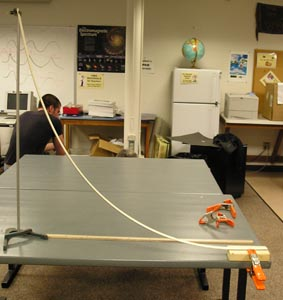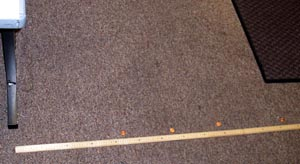Ball LauncherThe pvc ramp drops from the ringstand to the two-by-four clamped to the table.

Material

• A 6 foot length of 1 inch diameter PVC pipe
• A bandsaw
• a 6 inch piece of 2 by 4
• hotmelt glue gun
• a meterstick
• a table
• a C-clamp
• a ring stand 1 meter high and a test-tube clamp
• a marble
• 4 pennies
• two metersticks

Assembly

Use the bandsaw to cut the PVC tube into 4 strips.

Hot melt glue the PVC strips to the two-by-four

Use the C-clamp to attach the two-by-four to the edge of a table.

Attach the other end of the pvc to a ringstand 1 meter above the table.

To Do and NoticeCoins mark where the marbles hit.

Drop the marble from 80 cm above the two-by-four, 40 cm, 20 cm and 10 cm

Use the pennies to mark where the marble lands.

Repeat the drop to refine the positions of the pennies.

Measure the distance of each penny from the edge of the two-by-four.

Plot the distance of each penny versus the height of the dropped marble.

What's Going On?

Part 1:

When the marble drops down the ramp it's gravitational potential energy, mgh, is turned into kinetic energy.

If the marble did not roll but slid instead the equation would be:

mgh = 1/2 mv^2

where m is the mass of the marble and

where g is the acceleration of gravity,

h is the height of the drop of the marble in meters

and v is the velocity of the marble

This gives the velocity of the marble, which is horizontal, as it leaves the table.

v = (2gh)^0.5

(This is a lie see below for more truth)

It is true however that the mass of the marble doesn't matter and that the velocity is proportional to the square root of the velocity.

Part 2:

When a marble moving with velocity, v, leaves the table of height, H, and drops to the floor it always takes the same time to hit the floor, T. ( actually T = (2gH)^0.5)

The distance it travels D is proportional to the velocity of its launch, v.

D = v T

remember from part 1 that v = (h)^0.5

Here is the table of distances:

 h m D D m 0.1 1 0.44 0.2 1.4 0..66 0.4 2.0 0.88 0.8 2.8 1.22

h is the height in meters from which the marble is dropped

D is the distance that the marble travels. The middle row is relative distance.

Plot the distance of the marble versus the height of the drop.

Going Further

Some of the potential energy of the marble is converted into kinetic energy of rolling.

So the actual equation for the launch velocity is:

mgh = 1/2 mv^2 + 1/2 I w^2

where I is the moment of inertia of the marble which is 0.4 mr^2 with r the radius of the marble and where w is the angular velocity of the rolling marble in radians/s.

If a marble rolls without slipping then w = v/r

putting these together we get

mgh = 1/2 mv^2 + 1/2 0.4 mr^2 (v^2/r^2)

gh = 1/2 1.4 v^2

or v = (1/1.4)^0.5 * (2 gh)^0.5 = 0.85 (2gh)^0.5

The launch speed is 15% lower for a rolling object than for a sliding object.

 Scientific Explorations with Paul Doherty © 2004 8 October 2004